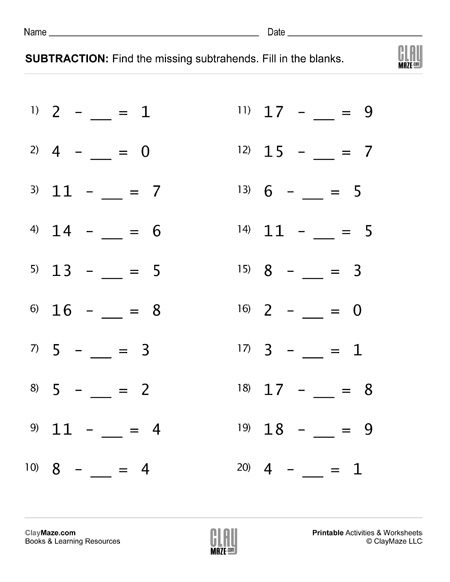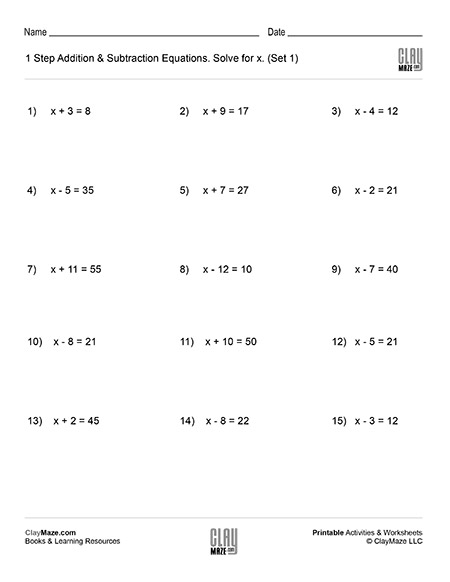Free Printable Worksheets Covering Addition and Subtraction with Whole Numbers and Fractions

## Subtraction Worksheet – Fill in the Blanks Subtraction Facts (Set 2)Fill in the blank subtraction worksheet. 20 problems. – with solutions...

## Subtraction Worksheet – Fill in the Blanks Subtraction Facts (Set 3)Worksheet for practice with subtraction facts. Fill in the blanks. Solutions included....

## Subtraction Worksheet – Fill in the Blanks Subtraction Facts (Set 4)Subtraction worksheet. Fill in the blanks with the correct subtrahends. Solutions are on page 2 of the pdf. Does your child need a little more Subtraction and Addition Practice? Try our workbook,...

## Algebra: 1 Step Addition & Subtraction Equations (Set 1)This worksheet contains problems that act as an introduction to algebra. The problems are 1 step addition and subtraction equations that ask the student to solve for x. – solutions included...

## Algebra: 1 Step Addition & Subtraction Equations (Set 2)Algebra introduction worksheet. 1 step addition and subtraction equations. – 15 problems with solutions...

## Algebra: 1 Step Addition & Subtraction Equations (Set 3)Intro algebra worksheet: Find the value of x for the problems listed. – 1 step addition and subtraction equations. – with solutions...

## Algebra: 1 Step Addition & Subtraction Equations (Set 4)Free algebra worksheet – 1 step addition and subtraction equations. – 15 problems per page – solutions included...